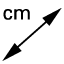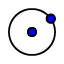# Investigation of Pi

Topic:
Geometry

## a.

What is the diameter of the circle? Select the distance tool, then click on point A followed by Point B.

b. What is the circumference of the circle? Again, select the distance tool, and then click anywhere along the circumference of the circle.

c. Using your calculator, divide the circumference you found above by your diameter. What do you notice?

## 2. Given the circle below, record the following measurements/answer the questions given below the image.

a. Just like questions 1 above, complete the following -Measure the diameter (Grab the distance tool,, then select point A and then point B. -Measure the circumference (again, grab the distance tool,, then click anywhere along the circumference -Using your own calculator, take the circumference and divide it by the diameter. Record your number below. How does it compare to the value of pi?

## a. Directions for constructing circle with a radius

Step 1. Select the Circle with Center Through Point Tool, click once with your mouse, and then drag your image. When you are happy with your circle, click again and your image will be complete. Step 2. Select the point tool, and then click anywhere along the circumference of your circle. Step 3. Select the line segment tool, click on your center point and than the radius.

b. Does the distance of our radius ever change inside the same circle? Now that you have your radius drawn inside of your circle, measure it's distance. Then, grab the move tool, and then click and drag the outside point on your circumference around the circle. Does your measurement change? What if you draw another radius?